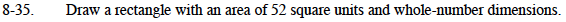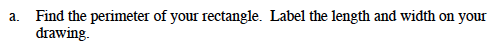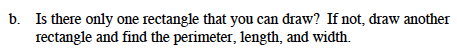### Home > MC1 > Chapter 8 > Lesson 8.2.2 > Problem8-35

8-35.Remember that the area of a rectangle is equal to length times width.
Try using division to find possible dimensions of a rectangle with the area of 52 square units.

1 by 52
2 by 26
3 by 13The perimeter of a rectangle is the distance around it.
Find the sum of all of the sides and you will have found the perimeter.
There are many possible rectangles.As you can see above, there are three possible rectangles.
Be sure your drawings are neat and don't forget to find their perimeters, lengths, and widths!